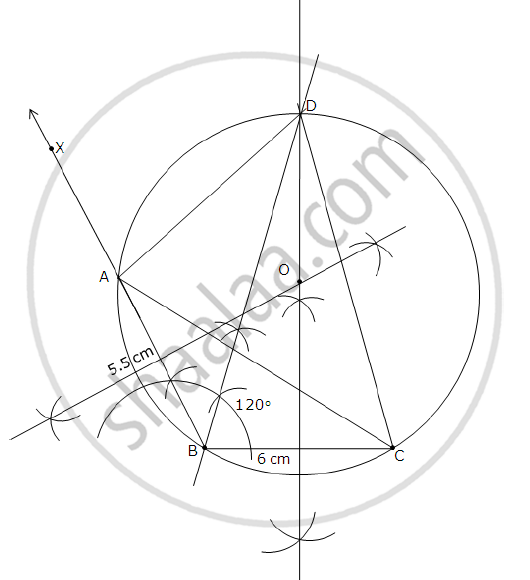# Construct a Triangle Abc in Which Base Bc = 6 Cm, Ab = 5.5 Cm and ∠Abc = 120°. Construct a Circle Circumscribing the Triangle Abc. Draw a Cyclic Quadrilateral Abcd So that D is Equidistant from B and C. - Mathematics

Construct a triangle ABC in which base BC = 6 cm, AB = 5.5 cm and ∠ABC = 120°.

Construct a circle circumscribing the triangle ABC.

Draw a cyclic quadrilateral ABCD so that D is equidistant from B and C.

#### Solution

Steps of construction:
1) Draw a line segment BC of length 6 cm.
2) At B, draw a ray BX making an angle of 120 with BC.
3) With B as centre and radius 5.5 cm, draw an arc to cut the ray BX at A. Join AC.
ΔABC will be obtained.
4) Draw the perpendicular bisectors of AB and BC to meet at point O.
5) With O as centre and radius OA, draw a circle. The circle will circumscribe ∠ABC.
6) Draw the angle bisector of ∠ABC.
7) The angle bisector of ∠ABC and the perpendicular bisector of line segment BC will
intersect at point D. Point D will be equidistant from points B and C.
8) Join AD and DC to obtain the required cyclic quadrilateral ABCD.Concept: Circumscribing and Inscribing a Circle on a Triangle
Is there an error in this question or solution?